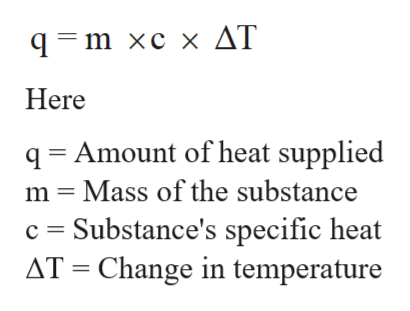# A chemical reaction takes place inside a flask submerged in a water bath. The water bath contains 6.50kg of water at 24.2°C. During the reaction 88.2kJ of heat flows out of the flask and into the bath.Calculate the new temperature of the water bath. You can assume the specific heat capacity of water under these conditions is 4.18·J·g−1K−1. Be sure your answer has the correct number of significant digits.

Question
564 views

A chemical reaction takes place inside a flask submerged in a water bath. The water bath contains 6.50kg of water at 24.2°C. During the reaction 88.2kJ of heat flows out of the flask and into the bath.Calculate the new temperature of the water bath. You can assume the specific heat capacity of water under these conditions is 4.18·J·g−1K−1. Be sure your answer has the correct number of significant digits.

check_circle

Step 1

The specific heat can be defined as the amount of heat per unit mass that is needed to raise the temperature of given substance by one degree Celsius.

The specific heat of water is 4.186 joule/gram °C. This can be used to calculate the heat required to raise the temperature for given substance.

Step 2

The relation between heat change and specific heat is shown below;help_outlineImage Transcriptionclosem xc x AT Here q Amount of heat supplied m Mass of the substance c Substance's specific heat AT Change in temperature fullscreen
Step 3

Given:

q = 88.2 kJ = 88.2 x 103 J
m = 6.50 kg = 6500 g
c = 4.186 j/g °C
Tf = final temperature =...

### Want to see the full answer?

See Solution

#### Want to see this answer and more?

Solutions are written by subject experts who are available 24/7. Questions are typically answered within 1 hour.*

See Solution
*Response times may vary by subject and question.
Tagged in

### Chemical Thermodynamics